E-mail：249324706@qq.com

# 钢材的基本公式

## 钢材的基本公式

2017-02-10 工程资料平台

 角钢=边长*边厚*0.015 焊管/无缝钢管=(外径-壁厚）*壁厚*0.02466 方管=边长*4*0.00785=周长除以3.14 矩形管=（长+宽）*2*壁厚*0.00785 扁钢=宽度*壁厚*0.00785 镀锌扁钢=宽度*壁厚*0.00785*1.06 板材=长度*宽度*厚度*0.00785 花纹板=[厚度*0.0785+0.3]*长度*宽度 六角钢=对边*对边距离*0.0065 八角钢=对边*对边距离*0.0065 不锈钢板=长度*宽度*厚度*7.93

 圆钢重量（公斤）=0.00617×直径×直径×长度 方钢重量（公斤）=0.00785×边宽×边宽×长度 六角钢重量（公斤）=0.0068×对边宽×对边宽×长度 八角钢重量（公斤）=0.0065×对边宽×对边宽×长度 螺纹钢重量（公斤）=0.00617×计算直径×计算直径×长度 角钢重量（公斤）=0.00785×（边宽+边宽-边厚）×边厚×长度 扁钢重量（公斤）=0.00785×厚度×边宽×长度 钢管重量（公斤）=0.02466×壁厚×（外径-壁厚）×长度

W（重量，kg ）=F(断面积 mm2)×L(长度，m)×ρ(密度，g/cm3)×1/1000

 名称（单位） 圆钢 盘条（kg/m） 计算公式 W= 0.006165 ×d×d 符号意义 d = 直径mm 计算举例 直径100 mm 的圆钢，求每m 重量。每m 重量= 0.006165 ×1002=61.65kg 钢材图片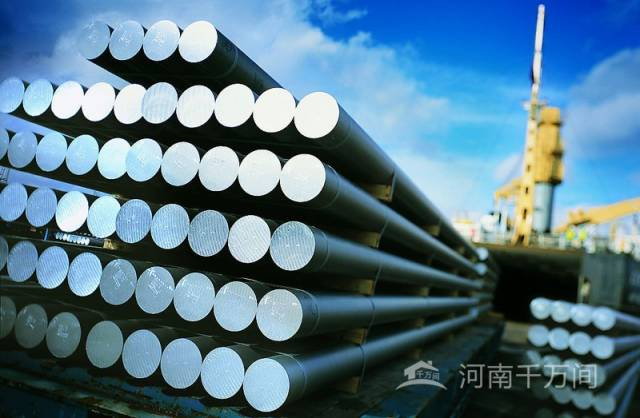名称（单位） 螺纹钢（kg/m） 计算公式 W= 0.00617 ×d×d 符号意义 d= 断面直径mm 计算举例 断面直径为12 mm 的螺纹钢，求每m 重量。每m 重量=0.00617 ×12 2=0.89kg 钢材图片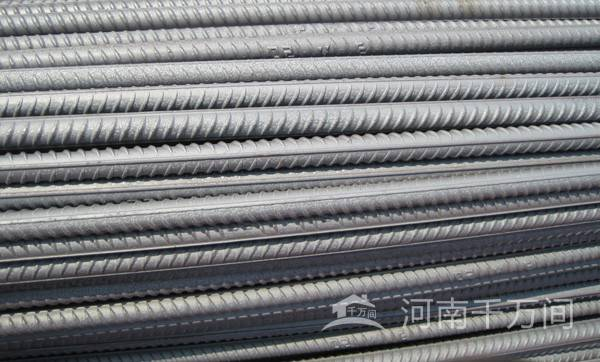名称（单位） 方钢（kg/m） 计算公式 W= 0.00785 ×a ×a 符号意义 a= 边宽mm 计算举例 边宽20 mm 的方钢，求每m 重量。每m 重量= 0.00785 ×202=3.14kg 钢材图片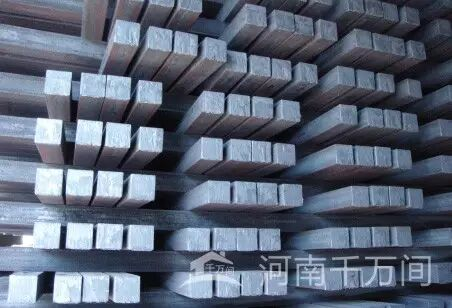名称（单位） 扁钢 （kg/m） 计算公式 W= 0.00785 ×b ×d 符号意义 b= 边宽mm   d= 厚mm 计算举例 边宽40 mm ，厚5mm 的扁钢，求每m 重量。每m 重量= 0.00785 ×40 ×5= 1.57kg 钢材图片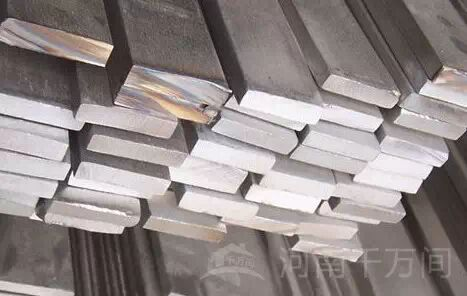名称（单位） 六角钢 （kg/m） 计算公式 W= 0.006798 ×s×s 符号意义 s= 对边距离mm 计算举例 对边距离50 mm 的六角钢，求每m 重量。每m 重量= 0.006798 ×502=17kg 钢材图片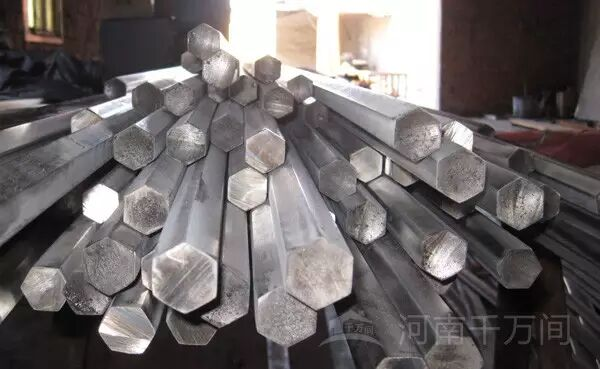名称（单位） 八角钢 （kg/m） 计算公式 W= 0.0065 ×s ×s 符号意义 s= 对边距离mm 计算举例 对边距离80 mm 的八角钢，求每m 重量。每m 重量= 0.0065 ×802=41.62kg

 名称（单位） 等边角钢 （kg/m） 计算公式 = 0.00785 ×[d （2b – d ）+0.215 （R2 – 2r 2 ）] 符号意义 b=边宽 d=边厚 R=内弧半径 r=端弧半径 计算举例 求20 mm×4mm 等边角钢的每m 重量。从冶金产品目录中查出4mm×20 mm等边角钢的R为3.5，r为1.2，则每m 重量=0.00785×[4×2×20–4）+0.215×（3.52–2×1.22）]=1.15kg 钢材图片

 名称（单位） 不等边角钢 （kg/m） 计算公式 W=0.00785×[d（B+b–d）+0.215（R2–2r2）] 符号意义 B=长边宽 b=短边宽 d=边厚 R=内弧半径 r=端弧半径 计算举例 求30mm×20mm×4mm 不等边角钢的每m 重量。从冶金产品目录中查出30×20×4不等边角钢的R为3.5，r 为1.2，则每m 重量= 0.00785×[4×（30+20 –4）+0.215×（3.52–2 ×1.22）]=1.46kg 钢材图片

 常 用 数 据 1 米(m)＝ 3.281 英尺 1 英寸＝ 25.4 毫米 1 磅＝ 0.4536 公斤 1 盎司＝ 28.3 克 1 公斤力＝ 9.81 牛顿 1 磅力＝4.45 牛顿 1 兆帕=145.161 磅 / 英寸 钢的比重(密度）： 7.8g/cm 不锈钢比重（密度）：7.78g/cm 锌比重（密度）： 7.05g/cm 铝的比重（密度）： 2.7g/cm

1、 角钢：每米重量=0.00785×（边宽+边宽—边厚）×边厚

2、 管材：每米重量=0.02466×壁厚×（外径—壁厚）

3、 圆钢：每m重量=0.00617×直径×直径   (螺纹钢和圆钢相同）

4、 方钢：每m重量=0.00786×边宽×边宽

5、 六角钢：每m重量=0.0068×对边直径×对边直径

6、 八角钢：每m重量=0.0065×直径×直径

7、 等边角钢：每m重量=边宽×边厚×0.015

8、 扁钢：每m重量=0.00785×厚度×宽度

9、 无缝钢管：每m重量=0.02466×壁厚×(外径-壁厚)

10、电焊钢：每m重量=无缝钢管

11、钢板：每㎡重量=7.85×厚度

12、黄铜管：每米重量=0.02670×壁厚×（外径-壁厚）

13、紫铜管：每米重量=0.02796×壁厚×（外径-壁厚)

14、铝花纹板：每平方米重量=2.96×厚度

15、有色金属密度：紫铜板8.9    黄铜板8.5     锌板7.2     铅板11.37

16、有色金属板材的计算公式为：每平方米重量=密度×厚度

17、方管:   每米重量=(边长+边长)×2×厚×0.00785

18、不等边角钢 ： 每米重量=0.00785×边厚(长边宽+短边宽--边厚)

19、工字钢   ：每米重量=0.00785×腰厚[高+f（腿宽-腰厚）]

20、槽钢： 每米重量=0.00785×腰厚[高+e（腿宽-腰厚）]

1、角钢、工字钢和槽钢的准确计算公式很繁，表列简式用于计算近似值。

2、f值：一般型号及带a的为3.34，带b的为2.65，带c的为2.26。

3、e值：一般型号及带a的为3.26，带b的为2.44，带c的为2.24。

4、各长度单位均为毫米（mm)

3投诉

TOP